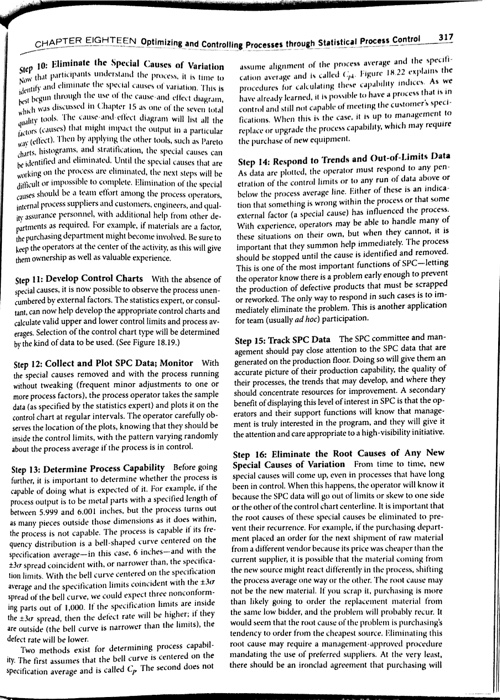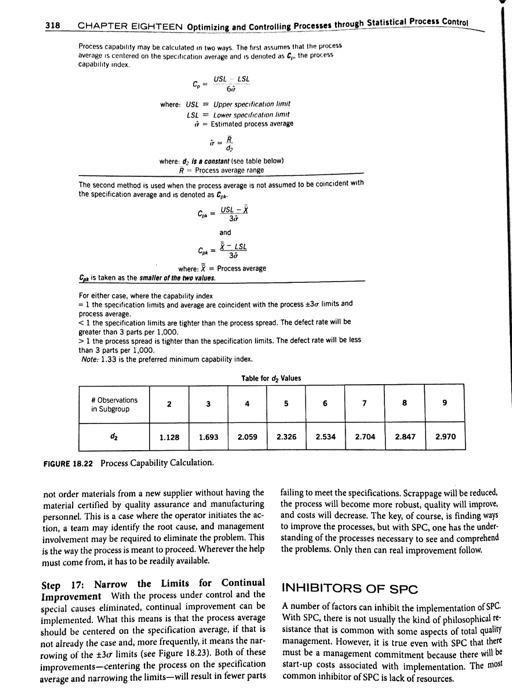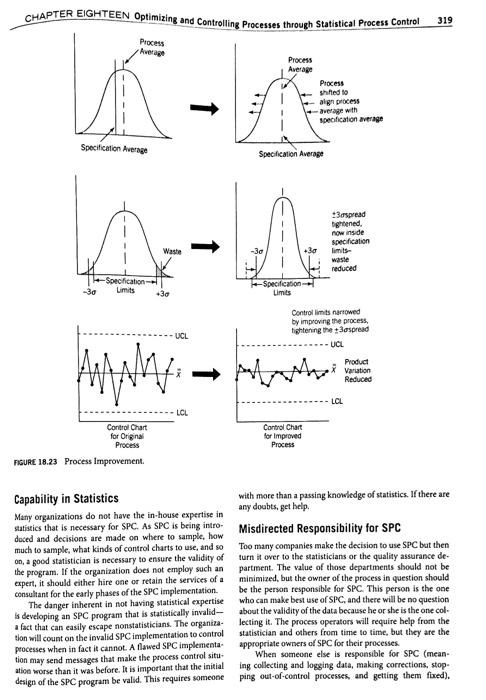# Calculate the Cp index to determine if Don Corleone’s pizza crust production system is capable of meeting its customers’ requirements. Briefly explain your findings

1. i) Process Capability (See Page 317 – 319 of Goetsch and Davis Chap. 18)
Formulas: (Do not use a d2 factor from fig. 18.22 ).
Instead, use the formula Cp = (USL – LSL)/6 σ        Note that σ is the standard deviation. USL and LSL are the customer’s specification limits.
Cpk is calculated in 2 ways:
Cpk = (USL – Xdouble bar)/ 3 σ   Note that X double bar is the process mean
Cpk = (X double bar – LSL)/ 3 σ

Customers of Don Corleone Pizza have specified that family-sized pizza crusts they order should be 28 to 32 centimeters in diameter. Sample data recently collected indicate that the crusts average 30 centimeters in diameter, with an estimated standard deviation sigma (σ) of 0.5 centimeters.

(i)    Calculate the Cp index to determine if Don Corleone’s pizza crust production system is capable of meeting its customers’ requirements. Briefly explain your findings

(ii)    Suppose that a new operator produces pizza crusts with a process average of 30.6 centimeters instead of 30 centimeters. Calculate the Cpk twice. How capable is the system capable of meeting its customers’ requirements? If not, what options does Don Corleone have to rectify the situation?In: Other